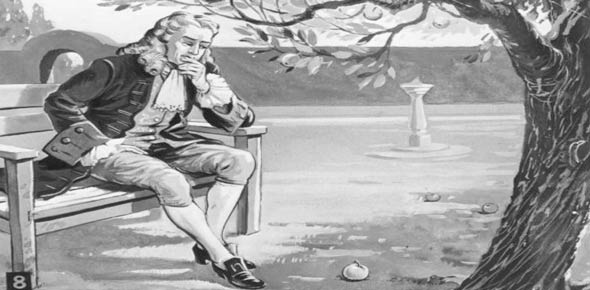# Newtons Laws

5 Questions | Total Attempts: 202SettingsRelated Topics
• 1.
Anything in motion/rest will reamine in motion/rest untill acted on by ________.
• A.

Gravity

• B.

An outside force

• C.

The four elements

• 2.
The thired law newton made was For every action there is an equal and opposite reaction.
• A.

True

• B.

False

• 3.
An objects force is dependet on it's ________and_______
• A.

Gravity and velocity

• B.

Speed and direction

• C.

Mass and acceleration

• D.

Time and distance

• E.

Speed and velocity

• 4.
The relationship between mass and inertia is:
• A.

Bigger the mass the smaller the inertia

• B.

Bigger the mass the bigger the inertia

• C.

Smaller the mass the bigger the inertia

• D.

Smaller the mass the smaller the inertia

• 5.
Air resistance is defined as wind blowing on an object in motion
• A.

True

• B.

False